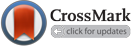Abd Almjeed, S. (2018). The Approximate Solution of the Fornberg-Whitham Equation by a Semi-Analytical Iterative Technique. , 36(2B), 120-123. doi: 10.30684/etj.36.2B.4
Sinan H. Abd Almjeed. "The Approximate Solution of the Fornberg-Whitham Equation by a Semi-Analytical Iterative Technique". , 36, 2B, 2018, 120-123. doi: 10.30684/etj.36.2B.4
Abd Almjeed, S. (2018). 'The Approximate Solution of the Fornberg-Whitham Equation by a Semi-Analytical Iterative Technique', , 36(2B), pp. 120-123. doi: 10.30684/etj.36.2B.4
Abd Almjeed, S. The Approximate Solution of the Fornberg-Whitham Equation by a Semi-Analytical Iterative Technique. , 2018; 36(2B): 120-123. doi: 10.30684/etj.36.2B.4